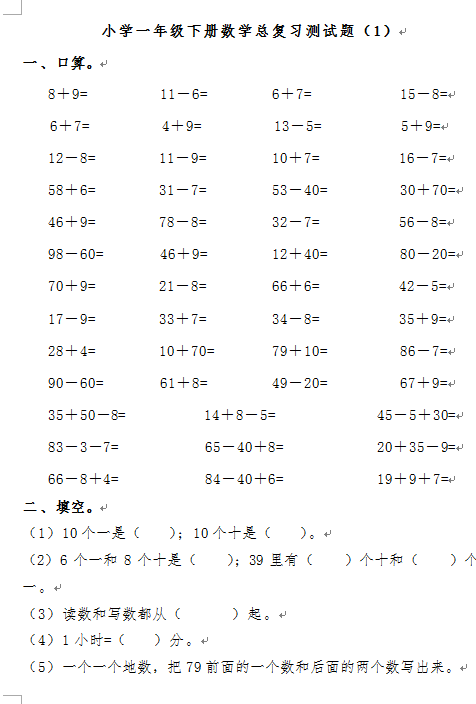8＋9=          11－6=         6＋7=            15－8=

6＋7=          4＋9=          13－5=           5＋9=

12－8=         11－9=         10＋7=           16－7=

【DOC文档85页】小学一年级下册数学复习测试题A4电子版资料_可直接打印_会员免费下载评论信息